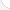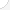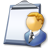Computer hardware engineers Description: Research, design, develop, and test computer or computer-related equipment for commercial, industrial, military, or scientific use. May supervise the manufacturing and installation of computer or computer-related equipment and components.Source: Bureau of Labor Statistics, U.S. Department of Labor, Occupational Outlook Handbook, 2008-09 Edition at http://www.bls.gov/OCO/Complete Job Profile: http://www.bls.gov/oco/ocos027.htmSalary: \$50,001 or more per yearComments:

There are 50 math topics Computer hardware engineers need to know.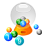Basic Math / Algebra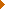FractionsDecimalsRatio and ProportionPercentCustomary MeasurementMetric MeasurementMeasurement ConversionBasic ProbabilityBasic StatisticsStatistical GraphingPowers and RootsOther Number BasesNegative NumbersScientific NotationBasic Problem Solving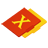First-Year AlgebraUsing FormulasLinear EquationsLinear InequalitiesLinear SystemsAlgebraic Representation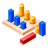GeometryBasic TerminologyAngle MeasurementCongruent TrianglesTriangle InequalitiesParallel LinesPythagorean TheoremRight Triangle TrigonometryCirclesAreaVolumeMake/Use 3D Drawings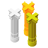Second-Year Algebra / TrigonometryFunctionsLogarithmsMatricesAdvanced StatisticsNon-Linear SystemsTrigonometric/Circular FunctionsGraphs of Trigonometric FunctionsTrigonometric IdentitiesTrigonometric Equations/Inverses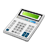Other TopicsCalculus and Higher MathBasic Calculator UseScientific Calculator UseComputer UseComputer ProgrammingGroup Problem SolvingMental MathInductive/Deductive ReasoningMath CommunicationsMathematical Modeling